### Table Output

The SEQTEST procedure displays the Design Information and Test Information tables by default.

#### Conditional Power

The Conditional Power Information table displays the following information under a hypothetical reference:

• stopping stage

• MLE, observed maximum likelihood estimate

• conditional power under the hypothetical reference

For a one-sided test, the power are derived under hypothetical references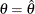and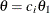, where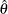is the observed statistic,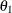is the alternative reference, and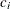are the values specified in the CREF= option. For a two-sided test, the power are derived under the hypothetical references,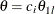, and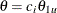, where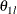is the lower alternative reference and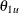is the upper alternative reference. The default is CREF= 0 0.5 1.0 1.5.

The Design Information table displays the design specifications and derived statistics. The derived Max Information (Percent Fixed-Sample) is the maximum information for the sequential design in percentage of the corresponding fixed-sample information.

The Null Ref ASN (Percent Fixed-Sample) is the average sample size required under the null hypothesis for the group sequential design in percentage of the corresponding fixed-sample design. Similarly, the Alt Ref ASN (Percent Fixed-Sample) is the average sample size required under the alternative reference for the group sequential design in percentage of the corresponding fixed-sample design.

#### Error Spending Information

The Error Spending Information table displays the following information at each stage:

• proportion of information

• actual information level, if the maximum information is either specified or derived

• cumulative error spending for each boundary

#### Parameter Estimates

The Parameter Estimates table displays the following information at the conclusion of a sequential trial:

• stopping stage

• parameter estimate

• median and confidence limits based on the specified ordering

• p-value for the hypothesisbased on the specified ordering

#### Powers and Expected Sample Sizes

The Powers and Expected Sample Sizes table displays the following information under each of the specified hypothetical references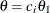, whereis the alternative reference andare values specified in the CREF= option.

• coefficientfor the hypothetical references. The value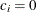corresponds to the null hypothesis and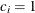corresponds to the alternative hypothesis

• power

• expected sample size, as percentage of fixed-sample size

For a one-sided design, the power and expected sample sizes under the hypothetical referencesare displayed.

For a two-sided symmetric design, the power and expected sample sizes under each of the hypothetical referencesare displayed, whereis the upper alternative reference.

For a two-sided asymmetric design, the power and expected sample sizes under each of the hypothetical referencesandare displayed, whereandare the lower and upper alternative references, respectively.

For a two-sided design, the power is the probability of correctly rejecting the null hypothesis for the correct alternative. Thus, under the null hypothesis, the displayed power corresponds to a one-sided Type I error probability level—that is, the lowerlevel or the upperlevel.

The expected sample size as a percentage of the corresponding fixed-sample design is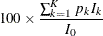where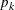is the stopping probability at stage k,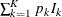is the expected information level, and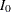is the information level for the fixed-sample design.

#### Predictive Power

The Predictive Power Information table displays the following information:

• stopping stage

• MLE, observed maximum likelihood estimate

• predictive power

#### Repeated Confidence Intervals

The Repeated Confidence Intervals table displays the following information for the observed statistic at each stage:

• information level

• parameter estimate

• rejection confidence limits. The null hypothesis is rejected for the upper alternative if the lower rejection confidence limit is greater than the null parameter value. Similarly, the null hypothesis is rejected for the lower alternative if the upper rejection confidence limit is less than the null parameter value.

• acceptance confidence limits. The upper alternative hypothesis is rejected if the upper acceptance confidence limit is less than the upper alternative value. Similarly, the lower alternative hypothesis is rejected if the lower acceptance confidence limit is greater than the upper alternative value. For a two-sided design, if both upper and lower alternative hypothesis are rejected, the null hypothesis is accepted.

#### Stopping Probabilities

The Expected Cumulative Stopping Probabilities table displays the following information under each of the specified hypothetical references, whereare values specified in the CREF= option, andis the alternative reference:

• coefficientfor the hypothetical references. The valuecorresponds to the null hypothesis, andcorresponds to the alternative hypothesis

• expected stopping stage

• source of the stopping probability: reject(with STOP=REJECT or STOP=BOTH), accept(with STOP=ACCEPT or STOP=BOTH), or either reject or accept(with STOP=BOTH)

• expected cumulative stopping probabilities at each stage

For a one-sided design, the expected cumulative stopping probabilities under the hypothetical referencesare displayed.

For a two-sided design, the expected cumulative stopping probabilities under each of the hypothetical referencesandare displayed, whereandare the lower and upper alternative references, respectively.

Note that for a symmetric two-sided design, only the expected cumulative stopping probabilities under the hypothetical referencesare derived.

The expected stopping stage is given by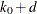and is derived from the expected information level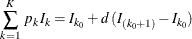whereis the stopping probability at stage k and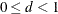.

For equally spaced information levels, the expected stopping stage is reduced to the weighted average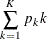#### Test Information

The Test Information table displays the following information at each stage:

• proportion of information

• actual information level, if the maximum information is available from the input BOUNDARY= data set

• alternative references with the specified statistic scale. If a p-value scale is specified, the standardized Z scale is used.

• boundary values with the specified statistic scale to reject or accept the null hypothesis

Note that implicitly, the test information table also contains variables for the boundary scale, stopping criterion, and type of alternative hypothesis. That is, if an ODS statement is used to save the table, the data set also contains the variables _Scale_ for the boundary scale, _Stop_ for the stopping criterion, and _ALT_ for the type of alternative hypothesis.

If the test variable is specified, the table also displays the following:

• test statistic

• resulting action of test statistic: continue to the next stage, accept the null hypothesis, or reject